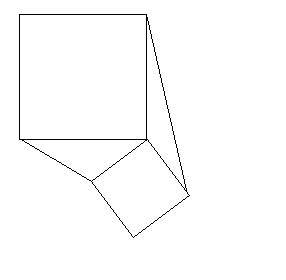You may also likeFixing It

A and B are two fixed points on a circle and RS is a variable diamater. What is the locus of the intersection P of AR and BS?OK! Now Prove It

Make a conjecture about the sum of the squares of the odd positive integers. Can you prove it?Summats Clear

Find the sum, f(n), of the first n terms of the sequence: 0, 1, 1, 2, 2, 3, 3........p, p, p +1, p + 1,..... Prove that f(a + b) - f(a - b) = ab.

Trig Rules OK

Age 16 to 18Challenge LevelDraw any two squares which meet at a common vertex $C$ and join the adjacent vertices to make two triangles $CAB$ and $CDE$.

Construct the perpendicular from $C$ to $AB$, (the altitude of the triangle). When you extend this line where does it cut $DE$?

Now bisect the line $AB$ to find the midpoint of this line $M$. Draw the median $MC$ of triangle $ABC$ and extend it to cut $DE$. What do you notice about the lines $MC$ and $DE$?

Will you get the same results about the two triangles formed if you draw squares of different sizes or at different angles to each other? Make a conjecture about the altitude of one of these triangles and prove your conjecture.

Thank you Geoff Faux for suggesting this problem.